## General Unpolarized Initial State

If we are just interested in the total decay rate, we can go further. The decay rate should not depend on the polarization of the initial state, based on the rotational symmetry of our theory. Usually we only want the total decay rate to some final state so we sum over polarizations of the photon, integrate over photon directions, and (eventually) sum over the differentof the final state atoms. We begin with a simple version of the total decay rate formula in the E1 approximation.Whereis the angle between the matrix element of the position vectorand the polarization vector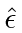. It is far easier to understand the sum over polarizations in terms of familiar vectors in 3-space than by using sums of Clebsch-Gordan coefficients.

Lets pick two transverse polarization vectors (to sum over) that form a right handed system with the direction of photon propagation.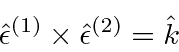The figure below shows the angles, basically picking the photon direction as the polar axis, and thedirection as what is usually called the x-axis.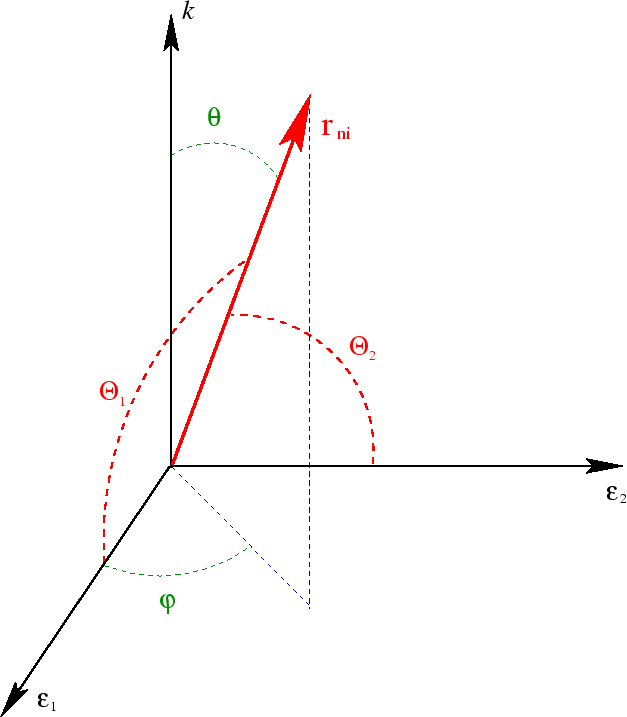The projection of the vectorinto the transverse plan gives a factor of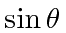. It is then easy to see thatThe sum ofover the two polarizations then just gives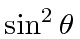. Therefore the decay rate becomesThis is now a very nice and simple result for the total decay rate of a state, summed over photon polarizations and integrated over photon direction.We still need to sum over the final atomic states as necessary. For the case of a transition in a single electron atom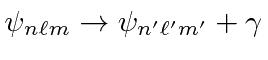, summed over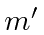, the properties of the Clebsch-Gordan coefficients can be used to show (See Merzbacher, second edition, page 467).The result is independent ofas we would expect from rotational symmetry.

As a simple check, lets recompute the 2p to 1s decay rate for hydrogen. We must choose thecase and.This is the same result we got in the explicit calculation.

Jim Branson 2013-04-22